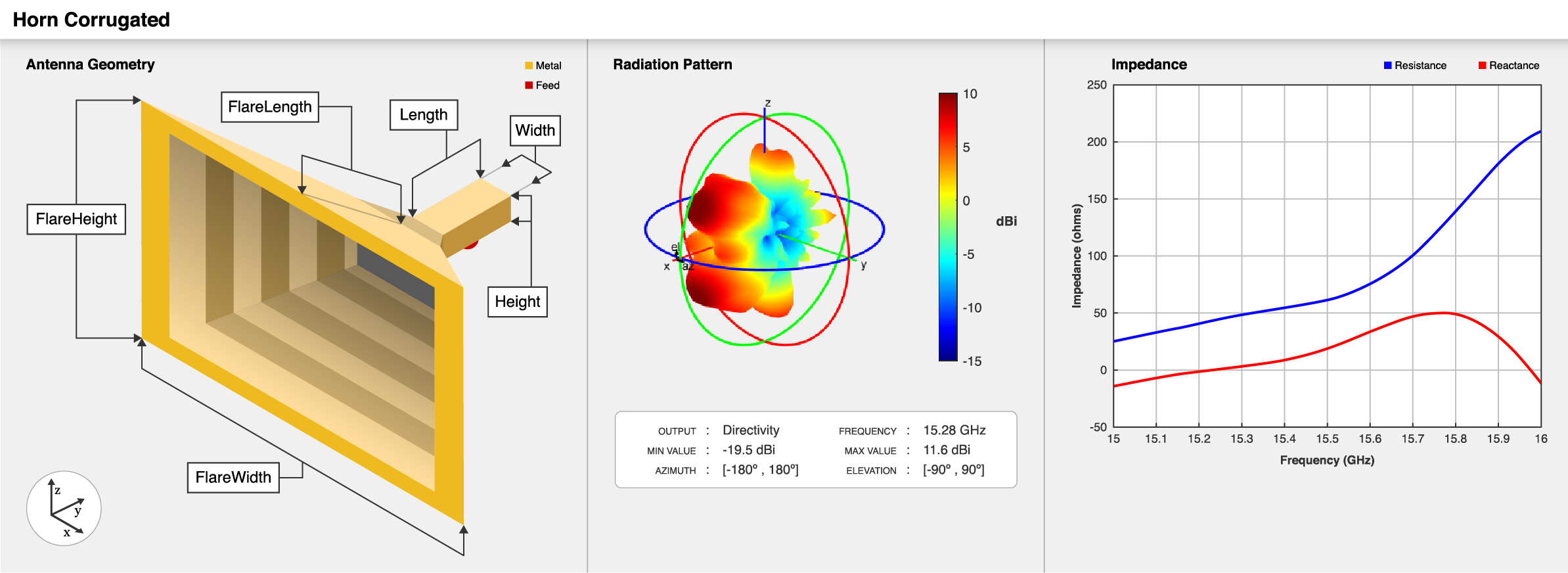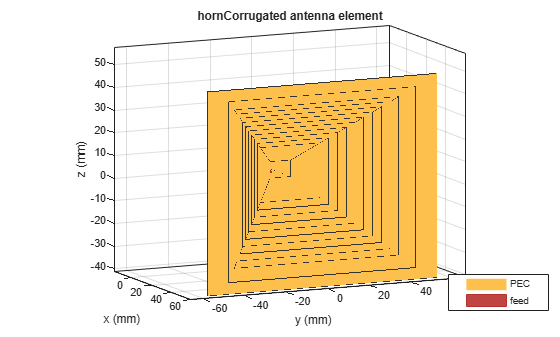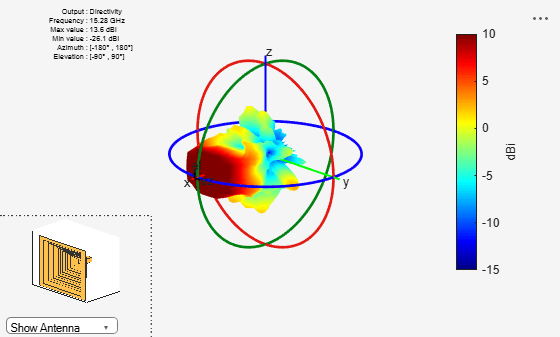# hornCorrugated

Create rectangular corrugated-horn antenna

Since R2020b

## Description

The `hornCorrugated` object creates a rectangular corrugated-horn antenna with grooves on the inner walls of the flare. These antennas provide spillover reduction and have beam symmetry and a low sidelobe level, so they are widely used as a feed in reflector antennas in broadcasting communications.## Creation

### Syntax

``ant = hornCorrugated``
``ant = hornCorrugated(Name,Value)``

### Description

example

````ant = hornCorrugated` creates a rectangular corrugated-horn antenna for a resonant frequency around 15 GHz. ```
````ant = hornCorrugated(Name,Value)` sets Properties using one or more name-value pairs. For example, ```ant = hornCorrugated('FlareLength', 0.045)``` creates a rectangular corrugated-horn antenna with the flare length of the horn set to 45 mm.```

## Properties

expand all

Flare length of the horn, specified as a positive scalar in meters.

Example: `'FlareLength',0.35`

Data Types: `double`

Flare width of the horn, specified as a positive scalar in meters.

Example: `'FlareWidth',0.2`

Data Types: `double`

Flare height of the horn, specified as a positive scalar in meters.

Example: `'FlareHeight',0.15`

Data Types: `double`

Length of the rectangular waveguide, specified as a positive scalar in meters.

Example: `'Length',0.09`

Data Types: `double`

Width of the rectangular waveguide, specified as a positive scalar in meters.

Example: `'Width',0.05`

Data Types: `double`

Height of the rectangular waveguide, specified as a positive scalar in meters.

Example: `'Height',0.0200`

Data Types: `double`

Height of the feed, specified as a positive scalar in meters.

Example: `'FeedHeight',0.0050`

Data Types: `double`

Width of the feed, specified as a positive scalar in meters.

Example: `'FeedWidth',5e-05`

Data Types: `double`

Signed distance of the feedpoint from the center of the ground plane, specified as a two-element vector in meters.

Example: `'FeedOffset',[–0.0070 0.01]`

Data Types: `double`

Distance between two successive corrugations, specified as a positive scalar in meters.

Example: `'Pitch',0.0060`

Example: `ant.Pitch = 0.0090`

Data Types: `double`

Distance of the first corrugation from the waveguide, specified as a positive scalar in meters.

Example: `'FirstCorrugatedDistance',0.0360`

Example: `ant.FirstCorrugatedDistance = 0.0190`

Data Types: `double`

Corrugation width, specified as a positive scalar in meters.

Example: `'CorrugateWidth',0.0058`

Example: `ant.CorrugateWidth = 0.0019`

Data Types: `double`

Corrugation depth, specified as a two-element vector in meters. The first element corresponds to the width along E-plane, and the second element corresponds to the width along the H-plane.

Example: `'CorrugateDepth',[0.006 0.0560]`

Example: `ant.CorrugateDepth = [0.0050 0.0790]`

Data Types: `double`

Type of the metal used as a conductor, specified as a metal material object. You can choose any metal from the `MetalCatalog` or specify a metal of your choice. For more information, see `metal`. For more information on metal conductor meshing, see Meshing.

Example: `m = metal('Copper'); 'Conductor',m`

Example: `m = metal('Copper'); ant.Conductor = m`

Tilt angle of the antenna, specified as a scalar or vector with each element unit in degrees. For more information, see Rotate Antennas and Arrays.

Example: `'Tilt',90`

Example: `ant.Tilt = 90`

Example: `'Tilt',[90 90]`,```'TiltAxis',[0 1 0;0 1 1]``` tilts the antenna at 90 degrees about the two axes defined by the vectors.

Data Types: `double`

Tilt axis of the antenna, specified as:

• Three-element vector of Cartesian coordinates in meters. In this case, each coordinate in the vector starts at the origin and lies along the specified points on the x-, y-, and z-axes.

• Two points in space, each specified as three-element vectors of Cartesian coordinates. In this case, the antenna rotates around the line joining the two points in space.

• A string input describing simple rotations around one of the principal axes, 'x', 'y', or 'z'.

Example: `'TiltAxis',[0 1 0]`

Example: `'TiltAxis',[0 0 0;0 1 0]`

Example: `ant.TiltAxis = 'Z'`

Lumped elements added to the antenna feed, specified as a `lumpedElement` object. You can add a load anywhere on the surface of the antenna. By default, the load is at the feed. For more information, see `lumpedElement`.

Example: `'Load',lumpedElement`. `lumpedElement` is the object for the load created using `lumpedElement`.

Example: `ant.Load = lumpedElement('Impedance',75)`

## Object Functions

 `show` Display antenna, array structures or shapes `axialRatio` Axial ratio of antenna `beamwidth` Beamwidth of antenna `charge` Charge distribution on antenna or array surface `current` Current distribution on antenna or array surface `design` Design prototype antenna or arrays for resonance around specified frequency `efficiency` Radiation efficiency of antenna `EHfields` Electric and magnetic fields of antennas; Embedded electric and magnetic fields of antenna element in arrays `impedance` Input impedance of antenna; scan impedance of array `mesh` Mesh properties of metal, dielectric antenna, or array structure `meshconfig` Change mesh mode of antenna structure `numCorrugationsToPitch` Calculate pitch for specified corrugations `optimize` Optimize antenna or array using SADEA optimizer `pattern` Radiation pattern and phase of antenna or array; Embedded pattern of antenna element in array `patternAzimuth` Azimuth pattern of antenna or array `patternElevation` Elevation pattern of antenna or array `rcs` Calculate and plot radar cross section (RCS) of platform, antenna, or array `returnLoss` Return loss of antenna; scan return loss of array `sparameters` Calculate S-parameter for antenna and antenna array objects `vswr` Voltage standing wave ratio of antenna

## Examples

collapse all

Create a default rectangular corrugated-horn antenna.

`ant = hornCorrugated`
```ant = hornCorrugated with properties: FlareLength: 0.0428 FlareWidth: 0.0900 FlareHeight: 0.0800 Length: 0.0229 Width: 0.0102 Height: 0.0075 FeedWidth: 8.0000e-05 FeedHeight: 0.0037 FeedOffset: [-0.0020 0] FirstCorrugateDistance: 0.0160 CorrugateDepth: [0.0050 0.0100] CorrugateWidth: 0.0030 Pitch: 0.0060 Conductor: [1x1 metal] Tilt: 0 TiltAxis: [1 0 0] Load: [1x1 lumpedElement] ```

View the antenna using the `show` function.

`show(ant)`Plot the radiation pattern of the antenna at a frequency 15.28 GHz.

```p = PatternPlotOptions('MagnitudeScale',[-15 10]); pattern(ant,15.28e9,'patternOptions',p)```Encinar, J., and J. Rebollar. “A Hybrid Technique for Analyzing Corrugated and Noncorrugated Rectangular Horns.” IEEE Transactions on Antennas and Propagation, vol. 34, no. 8, Aug. 1986, pp. 961–68.